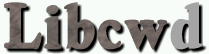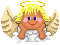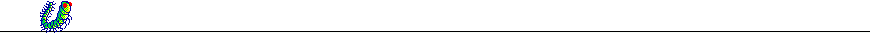The C++ Debugging Support Library By Carlo Wood, ©1999 - 2003.

## Tutorial 6: The debugging of dynamic memory allocations

### Introduction

For an introduction please read chapter Memory Allocation Debug Support: Introduction of the Reference Manual.

### Conventions

This tutorial will give a quick overview of the conventions that you have to follow to fully benefit from the support of libcwd for the debugging of dynamic memory allocations.

### Environment

First make sure that support is compiled in.  Check the headerfile libcwd/config.h and make sure that the macro `CWDEBUG_ALLOC` is set to 1.  If it is not defined, then you'll have to reconfigure, recompile and install libcwd.  Use ./configure --enable-alloc during configure.

You also want the macros `CWDEBUG_LOCATION` and `CWDEBUG_MAGIC` to be defined to 1.

There is no special header file needed.  You will need to include `"debug.h"` in the same way as is described in tutorial 5 and everything needed will be included from `libcwd/debug.h`.  In the remainder of this tutorial we will simple include `libcwd/debug.h` directly and not create any custom debug channels.

### The Allocated memory Overview

Libcwd does some basic checks on de-allocations and memory leaks (at the end of the program), but there is nothing to teach about that: you'll see what happens when you make a mistake.

However, at any moment in your program you can ask libcwd to print an overview of all memory allocations to a debug object (to channel `dc::malloc`) or to an arbitrary ostream.  All details of the Allocated memory Overview are described in chapter Overview Of Allocated Memory of the Reference Manual.

The following example program writes the Allocated memory Overview to the default debug object `libcw_do`:

Compile as: `g++ -DCWDEBUG amo.cc -lcwd -o amo`

```#include "sys.h"		// See tutorial 2.
#include "debug.h"

int main(void)
{
Debug( libcw_do.on() );
Debug( dc::malloc.on() );

Debug( list_allocations_on(libcw_do) );

return 0;
}
```

The output of this program is very simple,

```MALLOC  : Allocated memory: 0 bytes in 0 blocks.
```

because we didn't allocate any memory.

Now let us actually allocate some memory:

```#include "sys.h"		// See tutorial 2.
#include "debug.h"

int main(void)
{
Debug( libcw_do.on() );
Debug( dc::malloc.on() );

int* p = new int ;

Debug( list_allocations_on(libcw_do) );

return 0;
}
```

Also the call to `operator new[]` is written to debug channel MALLOC:

```MALLOC  : operator new[] (size = 400) = 0x804f310
MALLOC  : Allocated memory: 400 bytes in 1 blocks.
new[]     0x804f310             <unknown type>; (sz = 400)
```

The call to `list_allocations_on()` is responsible for the last two lines.

There is something missing however!  When we use `CWDEBUG_LOCATION` we expect source file and line number information of every memory allocation, and there is none.  In order to find out what is wrong, we also turn on debug channel` dc::bfd`:

```#include "sys.h"		// See tutorial 2.
#include "debug.h"

int main(void)
{
Debug( libcw_do.on() );
Debug( dc::malloc.on() );
Debug( dc::bfd.on() );

int* p = new int ;

Debug( list_allocations_on(libcw_do) );

return 0;
}
```

Which results in the following output:

```MALLOC  : operator new[] (size = 400) = <unfinished>
BFD     :     Loading debug symbols from /usr/local/lib/libcwd.so.0 (0x4001a000) ... done (1529 symbols)
BFD     :     Loading debug symbols from /usr/lib/libstdc++-libc6.1-2.so.3 (0x4006b000) ... done (1578 symbols)
BFD     :     Loading debug symbols from /lib/libm.so.6 (0x400b2000) ... done (1295 symbols)
BFD     :     Loading debug symbols from /lib/libc.so.6 (0x400cf000) ... done (4025 symbols)
BFD     :     Loading debug symbols from /usr/lib/libbfd-2.10.0.18.so (0x401c5000) ... done (1191 symbols)
BFD     :     Loading debug symbols from /lib/libdl.so.2 (0x4021c000) ... done (88 symbols)
BFD     :     Loading debug symbols from /lib/ld-linux.so.2 (0x40000000) ... done (293 symbols)
BFD     :     Warning: Address 0x804aa8e in section .text does not have a line number, perhaps the unit containing the function
`main' wasn't compiled with flag -g
MALLOC  : <continued> 0x804e898
MALLOC  : Allocated memory: 400 bytes in 1 blocks.
new[]     0x804e898             <unknown type>; (sz = 400)
```

• The debugging symbols are loaded the first time an address is resolved, interrupting the debug output of the first allocation.
• The allocation is done somewhere inside a function `main` but no Source-file:Line-number Information information is found.
• Likely the 'Loading debug symbols from..' is done before the application reaches `main()` and is hence invisible (because the debug object, `libcw_do`, is still turned off).  You can force libcwd to print it nevertheless by setting the environment variable `LIBCWD_PRINT_LOADING`.
 And indeed, we forgot to compile amo.cc with -gWhen we compile correctly, using `g++ -g -DCWDEBUG amo.cc -lcwd -o amo`, the output of the program becomes:

```MALLOC  : operator new[] (size = 400) = 0x804f208
MALLOC  : Allocated memory: 400 bytes in 1 blocks.
new[]     0x804f208               amo.cc:9    <unknown type>; (sz = 400)
```

As you can see, the type of the object for which the memory was allocated is still unknown.  You can make the Allocated memory Overview better surveyable by adding a «tag» for every allocation that your program is doing:

```#include "sys.h"		// See tutorial 2.
#include "debug.h"

int main(void)
{
Debug( libcw_do.on() );
Debug( dc::malloc.on() );

int* p = new int ;
AllocTag(p, "A test array");

Debug( list_allocations_on(libcw_do) );

return 0;
}
```

This results in the output

```MALLOC  : operator new[] (size = 400) = 0x804f7a8
MALLOC  : Allocated memory: 400 bytes in 1 blocks.
new[]     0x804f7a8               amo.cc:9    int; (sz = 400)  A test array
```

The second parameter of `AllocTag()` may be anything that can be written to an ostream (like is the case for `Dout()`).  However, it is only processed once: the first time it is called.  This allows to even add an `AllocTag()` at places where a low cpu usage is important and/or in loops that allocate a very large number of memory blocks (the comment is only stored once).

Consider the following code:

```#include "sys.h"		// See tutorial 2.
#include "debug.h"

int main(void)
{
Debug( libcw_do.on() );
Debug( dc::malloc.on() );

int* p;
for(int i = 0; i < 4; ++i)
{
p[i] = new int ;
AllocTag(p[i], "Test array number " << i);	// This won't work
}

Debug( list_allocations_on(libcw_do) );

return 0;
}
```

The Allocated memory Overview will show four times the same tag:

```MALLOC  : operator new[] (size = 400) = 0x804f8d0
MALLOC  : operator new[] (size = 400) = 0x8137c80
MALLOC  : operator new[] (size = 400) = 0x8138028
MALLOC  : operator new[] (size = 400) = 0x81383d0
MALLOC  : Allocated memory: 1600 bytes in 4 blocks.
new[]     0x81383d0               amo.cc:12   int; (sz = 400)  Test array number 0
new[]     0x8138028               amo.cc:12   int; (sz = 400)  Test array number 0
new[]     0x8137c80               amo.cc:12   int; (sz = 400)  Test array number 0
new[]     0x804f8d0               amo.cc:12   int; (sz = 400)  Test array number 0
```

If you don't care about the extra memory and cpu usage, you can also use `AllocTag_dynamic_description()`, which will work.

```#include "sys.h"		// See tutorial 2.
#include "debug.h"

int main(void)
{
Debug( libcw_do.on() );
Debug( dc::malloc.on() );

int* p;
for(int i = 0; i < 4; ++i)
{
p[i] = new int ;
AllocTag_dynamic_description(p[i], "Test array number " << i);	// This will work
}

Debug( list_allocations_on(libcw_do) );

return 0;
}
```

gives as result

```MALLOC  : operator new[] (size = 400) = 0x804f968
MALLOC  : operator new[] (size = 400) = 0x8137d70
MALLOC  : operator new[] (size = 400) = 0x8138290
MALLOC  : operator new[] (size = 400) = 0x804f6f8
MALLOC  : Allocated memory: 1600 bytes in 4 blocks.
new[]     0x804f6f8               amo.cc:12   int; (sz = 400)  Test array number 3
new[]     0x8138290               amo.cc:12   int; (sz = 400)  Test array number 2
new[]     0x8137d70               amo.cc:12   int; (sz = 400)  Test array number 1
new[]     0x804f968               amo.cc:12   int; (sz = 400)  Test array number 0
```

Often just the type of an object tells you enough about what it is.  In that case you can omit the comment completely and simply use `AllocTag1(p)`.  Or, if you don't need any `operator<<`, you can use `AllocTag2(p, "Some string constant here")`.  Finally, you can use the macro `NEW` to catch the type of an allocation as if you did a `new` followed by a `AllocTag1(p)`:

```#include "sys.h"		// See tutorial 2.
#include "debug.h"

int main(void)
{
Debug( libcw_do.on() );
Debug( dc::malloc.on() );

int* p = NEW( int  );

Debug( list_allocations_on(libcw_do) );

return 0;
}
```

would output

```MALLOC  : operator new[] (size = 400) = 0x804f4f0
MALLOC  : Allocated memory: 400 bytes in 1 blocks.
new[]     0x804f4f0               amo.cc:9    int; (sz = 400)
```